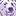# New to Qlik Sense

If you’re new to Qlik Sense, start with this Discussion Board and get up-to-speed quickly.

Announcements
QlikWorld 2023, a live, in-person thrill ride. April 17 - 20, 2023, in Las Vegas! REGISTER TODAY
cancel
Showing results for
Did you mean:Partner - Contributor

## P&L Issue with variable targets

Hi there,

I am trying to build a P&L which has several calculated targets, based on actual income (e.g. FoodCost's target should be 42% of actual income).

I've managed to get all targets value to appear correctly for ExpenseType=(Income,Fixed), however, I can't manage to get the variable expenses to show correctly.

For the Target calculation I tried this:

If (
TargetType='Absoloute',
Sum(TargetValue),
Sum(TargetValue)*Sum({<Expense={'Income'}>}ActualValue)*-1
)

This statement results in showing 0.00 for the target value.

Attached are the excel

2 RepliesCreator

Could it be because the first row is BOTH TargetType='Absoloute' and Expense={'Income'}, so the if-statement skips that when you try to calculate the else value "Sum(TargetValue)*Sum({<Expense={'Income'}>}ActualValue)*-1"?

What happens if you try

If (
TargetType='Absoloute' and Expense<>'Income',
Sum(TargetValue),
Sum(TargetValue)*Sum({<Expense={'Income'}>}ActualValue)*-1
)Partner - Contributor
Author

Thanks, Anseglko, but it didn't work.

My relative target for Expense=FoodCost is 42% of actual income for each month.

So, if the actual income in March is 65,340\$, the FoodCost target for that month should be: 27,442\$. This calculation should be done for each month which has actual income.